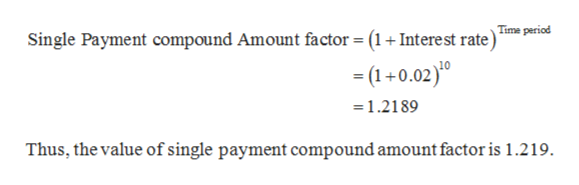# 1. What is the Single Payment Compound Amount factor for an interest rate of 2% over 10 years? 2. What is the Uniform Gradient Future Worth factor for an interest rate of 10% over 10 years? 3. What is the Uniform Series Present Worth factor for an interest rate of 5.5% over 20 years? 4. A bank pays 3% interest per year (compounded annually).a. To what amount will a \$5,000 deposit grow if left in the bank for 10 years?b. Draw the Cash Flow Diagram for this problem.

Question
3 views

1. What is the Single Payment Compound Amount factor for an interest rate of 2% over 10 years?

2. What is the Uniform Gradient Future Worth factor for an interest rate of 10% over 10 years?

3. What is the Uniform Series Present Worth factor for an interest rate of 5.5% over 20 years?

4. A bank pays 3% interest per year (compounded annually).

a. To what amount will a \$5,000 deposit grow if left in the bank for 10 years?

b. Draw the Cash Flow Diagram for this problem.

check_circle

Step 1

1.

Single Payment compound amount:...help_outlineImage TranscriptioncloseSingle Payment compound Amount factor = (1+ Interest rate) m p = 1+0.02)0 1.2189 Thus, the value of single payment compound amount factor is 1.219 fullscreen

### Want to see the full answer?

See Solution

#### Want to see this answer and more?

Solutions are written by subject experts who are available 24/7. Questions are typically answered within 1 hour.*

See Solution
*Response times may vary by subject and question.
Tagged in